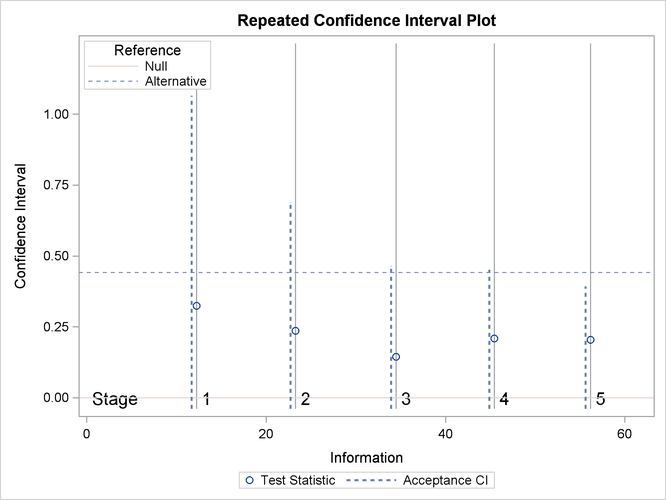### Example 84.5 Comparing Two Proportions with a Log Odds Ratio Test

This example compares two binomial proportions by using a log odds ratio statistic in a five-stage group sequential test. A clinic is studying the effect of vitamin C supplements in treating flu symptoms. The study consists of patients in the clinic who exhibit the first sign of flu symptoms within the last 24 hours. These patients are randomly assigned to either the control group (which receives placebo pills) or the treatment group (which receives large doses of vitamin C supplements). At the end of a five-day period, the flu symptoms of each patient are recorded.

Suppose that you know from past experience that flu symptoms disappear in five days for 60% of patients who experience flu symptoms. The clinic would like to detect a 70% symptom disappearance with a high probability. A test that compares the proportions directly specifies the null hypothesis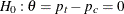with a one-sided alternative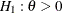and a power of 0.90 at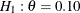, where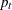and
are the proportions of symptom disappearance in the treatment group and control group, respectively. An alternative trial tests an equivalent hypothesis by using the log odds ratio statistics: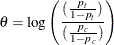Then the null hypothesis is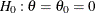and the alternative hypothesis is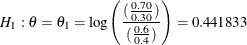The following statements invoke the SEQDESIGN procedure and request a five-stage group sequential design by using an error spending function method for normally distributed statistics. The design uses a two-sided alternative hypothesis with early stopping to reject the null hypothesis.

ods graphics on;
proc seqdesign altref=0.441833
boundaryscale=mle
;
OneSidedErrorSpending: design method=errfuncpow
nstages=5
alt=upper
stop=accept
alpha=0.025;
samplesize model=twosamplefreq( nullprop=0.6 test=logor);
ods output Boundary=Bnd_CSup;
run;
ods graphics off;


The ODS OUTPUT statement with the BOUNDARY=BND_CSUP option creates an output data set named BND_CSUP which contains the resulting boundary information for the subsequent sequential tests.

The Design Information table in Output 84.5.1 displays design specifications and derived statistics. With the specified alternative reference, the maximum information 56.30934 is derived.

The SEQDESIGN Procedure
Design: OneSidedErrorSpending

Statistic Distribution Normal
Boundary Scale MLE
Alternative Hypothesis Upper
Early Stop Accept Null
Method Error Spending
Boundary Key Both
Alternative Reference 0.441833
Number of Stages 5
Alpha 0.025
Beta 0.1
Power 0.9
Max Information (Percent of Fixed Sample) 104.6166
Max Information 56.30934
Null Ref ASN (Percent of Fixed Sample) 57.21399
Alt Ref ASN (Percent of Fixed Sample) 102.1058

The Boundary Information table in Output 84.5.2 displays information level, alternative reference, and boundary values at each stage. With the specified BOUNDARYSCALE=MLE option, the procedure displays the output boundaries in terms of the MLE scale.

Output 84.5.2: Boundary Information

Boundary Information (MLE Scale)
Null Reference = 0
_Stage_   Alternative Boundary Values
Information Level Reference Upper
Proportion Actual N Upper Beta
1 0.2000 11.26187 201.1048 0.44183 -0.34844
2 0.4000 22.52374 402.2096 0.44183 -0.02262
3 0.6000 33.7856 603.3144 0.44183 0.11527
4 0.8000 45.04747 804.4192 0.44183 0.19708
5 1.0000 56.30934 1005.524 0.44183 0.25345

With ODS Graphics enabled, a detailed boundary plot with the rejection and acceptance regions is displayed, as shown in Output 84.5.3.

Output 84.5.3: Boundary Plot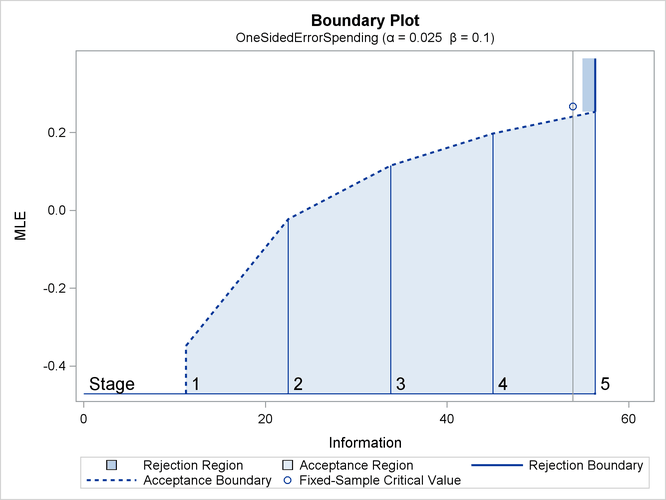With the SAMPLESIZE statement, the Sample Size Summary table in Output 84.5.4 displays the parameters for the sample size computation.

Output 84.5.4: Sample Size Summary

Sample Size Summary
Test Two-Sample Proportions
Null Proportion 0.6
Proportion (Group A) 0.7
Test Statistic Log Odds Ratio
Reference Proportions Alt Ref
Max Sample Size 1005.524
Expected Sample Size (Null Ref) 549.9132
Expected Sample Size (Alt Ref) 981.3914

The Sample Sizes table in Output 84.5.5 displays the required sample sizes for the group sequential clinical trial.

Output 84.5.5: Required Sample Sizes

Sample Sizes (N)
Two-Sample Log Odds Ratio Test for Proportion Difference
_Stage_ Fractional N Ceiling N
N N(Grp 1) N(Grp 2) Information N N(Grp 1) N(Grp 2) Information
1 201.10 100.55 100.55 11.2619 202 101 101 11.3120
2 402.21 201.10 201.10 22.5237 404 202 202 22.6240
3 603.31 301.66 301.66 33.7856 604 302 302 33.8240
4 804.42 402.21 402.21 45.0475 806 403 403 45.1360
5 1005.52 502.76 502.76 56.3093 1006 503 503 56.3360

Thus, 101 new patients are needed in each group at stages 1, 2, and 4, and 100 new patients are needed in each group at stages 3 and 5. Suppose that 101 patients are available in each group at stage 1. Output 84.5.6 lists the 10 observations in the data set count_1.

Output 84.5.6: Clinical Trial Data

 First 10 Obs in the Trial Data

Obs TrtGrp Resp
1 Control 1
2 C_Sup 0
3 Control 0
4 C_Sup 1
5 Control 1
6 C_Sup 1
7 Control 1
8 C_Sup 0
9 Control 0
10 C_Sup 1

The TrtGrp variable is a grouping variable with the value Control for a patient in the placebo control group and the value C_Sup for a patient in the treatment group who receives vitamin C supplements. The Resp variable is an indicator variable with the value 1 for a patient without flu symptoms after five days and the value 0 for a patient with flu symptoms after five days.

The following statements use the LOGISTIC procedure to compute the log odds ratio statistic and its associated standard error at stage 1:

proc logistic data=CSup_1 descending;
class TrtGrp / param=ref;
model Resp= TrtGrp;
ods output ParameterEstimates=Parms_CSup1;
run;


The DESCENDING option is used to reverse the order for the response levels, so the LOGISTIC procedure is modeling the probability that Resp = 1.

The following statements create and display (in Output 84.5.7) the data set for the log odds ratio statistic and its associated standard error:

data Parms_CSup1;
set Parms_CSup1;
if Variable='TrtGrp' and ClassVal0='C_Sup';
_Scale_='MLE';
_Stage_= 1;
keep _Scale_ _Stage_ Variable Estimate StdErr;
run;

proc print data=Parms_CSup1;
title 'Statistics Computed at Stage 1';
run;


Output 84.5.7: Statistics Computed at Stage 1

 Statistics Computed at Stage 1

Obs Variable Estimate StdErr _Scale_ _Stage_
1 TrtGrp 0.3247 0.2856 MLE 1

The following statements invoke the SEQTEST procedure to test for early stopping at stage 1:

ods graphics on;
proc seqtest Boundary=Bnd_CSup
Parms(Testvar=TrtGrp)=Parms_CSup1
boundarykey=both
boundaryscale=mle
;
ods output test=Test_CSup1;
run;
ods graphics off;


The BOUNDARY= option specifies the input data set that provides the boundary information for the trial at stage 1, which was generated in the SEQDESIGN procedure. The PARMS=PARMS_CSUP1 option specifies the input data set PARMS_CSUP1 that contains the test statistic and its associated standard error at stage 1, and the TESTVAR=TRTGRP option identifies the test variable TRTGRP in the data set.

If the computed information level for stage 1 is not the same as the value provided in the BOUNDARY= data set, the INFOADJ=PROP option (which is the default) proportionally adjusts the information levels at future interim stages from the levels provided in the BOUNDARY= data set. The ERRSPENDADJ=ERRFUNCPOW option adjusts the boundaries with the updated error spending values generated from the power error spending function. The BOUNDARYKEY=BOTH option maintains both theandlevels. The BOUNDARYSCALE=MLE option displays the output boundaries in terms of the MLE scale.

The ODS OUTPUT statement with the TEST=TEST_CSUP1 option creates an output data set named TEST_CSUP1 which contains the updated boundary information for the test at stage 1. The data set also provides the boundary information that is needed for the group sequential test at the next stage.

The Design Information table in Output 84.5.8 displays design specifications. With the specified BOUNDARYKEY=BOTH option, the information levels and boundary values at future stages are modified to maintain both theandlevels.

The SEQTEST Procedure

BOUNDARY Data Set WORK.BND_CSUP
Data Set WORK.PARMS_CSUP1
Statistic Distribution Normal
Boundary Scale MLE
Alternative Hypothesis Upper
Early Stop Accept Null
Number of Stages 5
Alpha 0.025
Beta 0.1
Power 0.9
Max Information (Percent of Fixed Sample) 104.6673
Max Information 56.3361718
Null Ref ASN (Percent of Fixed Sample) 57.02894
Alt Ref ASN (Percent of Fixed Sample) 102.1369

The Test Information table in Output 84.5.9 displays the boundary values for the test statistic with the specified MLE scale. With the INFOADJ=PROP option (which is the default), the information levels at future interim stages are derived proportionally from the observed information at stage 1 and the information levels in the BOUNDARY= data set.

Since the information level at stage 1 is derived from the PARMS= data set and other information levels are not specified, equal increments are used at remaining stages. At stage 1, the MLE statistic 0.32474 is greater than the corresponding upperboundary value –0.29906, so the sequential test continues to the next stage.

Output 84.5.9: Sequential Tests

Test Information (MLE Scale)
Null Reference = 0
_Stage_   Alternative Boundary Values Test
Information Level Reference Upper TrtGrp
Proportion Actual Upper Beta Estimate Action
1 0.2176 12.26014 0.44183 -0.29906 0.32474 Continue
2 0.4132 23.27914 0.44183 -0.01067 .
3 0.6088 34.29815 0.44183 0.11942 .
4 0.8044 45.31716 0.44183 0.19829 .
5 1.0000 56.33617 0.44183 0.25325 .

With ODS Graphics enabled, a boundary plot with the boundary values and test statistics is displayed, as shown in Output 84.5.10. As expected, the test statistic is in the continuation region.

Output 84.5.10: Sequential Test Plot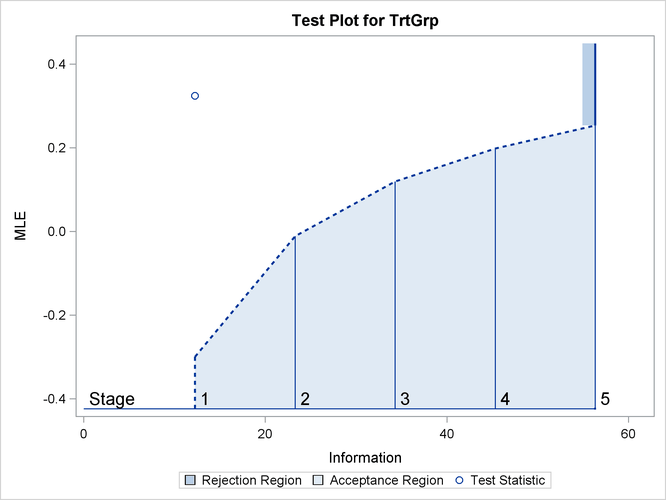The following statements use the LOGISTIC procedure to compute the log odds ratio statistic and its associated standard error at stage 2:

proc logistic data=CSup_2 descending;
class TrtGrp / param=ref;
model Resp= TrtGrp;
ods output ParameterEstimates=Parms_CSup2;
run;


The following statements create and display (in Output 84.5.11) the data set for the mean positive response and its associated standard error at stage 2:

data Parms_CSup2;
set Parms_CSup2;
if Variable='TrtGrp' and ClassVal0='C_Sup';
_Scale_='MLE';
_Stage_= 2;
keep _Scale_ _Stage_ Variable Estimate StdErr;
run;
proc print data=Parms_CSup2;
title 'Statistics Computed at Stage 2';
run;


Output 84.5.11: Statistics Computed at Stage 2

 Statistics Computed at Stage 2

Obs Variable Estimate StdErr _Scale_ _Stage_
1 TrtGrp 0.2356 0.2073 MLE 2

The following statements invoke the SEQTEST procedure to test for early stopping at stage 2:

proc seqtest Boundary=Test_CSup1
Parms( testvar=TrtGrp)=Parms_CSup2
boundarykey=both
boundaryscale=mle
;
ods output Test=Test_CSup2;
run;


The BOUNDARY= option specifies the input data set that provides the boundary information for the trial at stage 2, which was generated by the SEQTEST procedure at the previous stage. The PARMS= option specifies the input data set that contains the test statistic and its associated standard error at stage 2, and the TESTVAR= option identifies the test variable in the data set.

The ODS OUTPUT statement with the TEST=CSUP_LDL2 option creates an output data set named CSUP_LDL2 which contains the updated boundary information for the test at stage 2. The data set also provides the boundary information that is needed for the group sequential test at the next stage.

The Test Information table in Output 84.5.12 displays the boundary values for the test statistic with the specified MLE scale. The test statistic 0.2356 is greater than the corresponding upperboundary value –0.01068, so the sequential test continues to the next stage.

Output 84.5.12: Sequential Tests

The SEQTEST Procedure

Test Information (MLE Scale)
Null Reference = 0
_Stage_   Alternative Boundary Values Test
Information Level Reference Upper TrtGrp
Proportion Actual Upper Beta Estimate Action
1 0.2176 12.26014 0.44183 -0.29906 0.32474 Continue
2 0.4132 23.27916 0.44183 -0.01068 0.23560 Continue
3 0.6088 34.29799 0.44183 0.11942 .
4 0.8044 45.31681 0.44183 0.19829 .
5 1.0000 56.33563 0.44183 0.25325 .

Similar results are found at stages 3 and stage 4, so the trial continues to the final stage. The following statements use the LOGISTIC procedure to compute the log odds ratio statistic and its associated standard error at stage 5:

proc logistic data=CSup_5 descending;
class TrtGrp / param=ref;
model Resp= TrtGrp;
ods output ParameterEstimates=Parms_CSup5;
run;


The following statements create and display (in Output 84.5.13) the data set for the log odds ratio statistic and its associated standard error at stage 5:

data Parms_CSup5;
set Parms_CSup5;
if Variable='TrtGrp' and ClassVal0='C_Sup';
_Scale_='MLE';
_Stage_= 5;
keep _Scale_ _Stage_ Variable Estimate StdErr;
run;

proc print data=Parms_CSup5;
title 'Statistics Computed at Stage 5';
run;


Output 84.5.13: Statistics Computed at Stage 5

 Statistics Computed at Stage 5

Obs Variable Estimate StdErr _Scale_ _Stage_
1 TrtGrp 0.2043 0.1334 MLE 5

The following statements invoke the SEQTEST procedure to test for the hypothesis at stage 5:

ods graphics on;
proc seqtest Boundary=Test_CSup4
Parms( testvar=TrtGrp)=Parms_CSup5
boundaryscale=mle
cialpha=.025
rci
plots=rci
;
run;
ods graphics off;


The BOUNDARY= option specifies the input data set that provides the boundary information for the trial at stage 5, which was generated by the SEQTEST procedure at the previous stage. The PARMS= option specifies the input data set that contains the test statistic and its associated standard error at stage 5, and the TESTVAR= option identifies the test variable in the data set. By default (or equivalently if you specify BOUNDARYKEY=ALPHA), the boundary value at stage 5 is derived to maintain thelevel.

The Test Information table in Output 84.5.14 displays the boundary values for the test statistic with the specified MLE scale. The test statistic 0.2043 is less than the corresponding upperboundary 0.25375, so the sequential test stops to accept the null hypothesis. That is, there is no reduction in duration of symptoms for the group receiving vitamin C supplements.

Output 84.5.14: Sequential Tests

The SEQTEST Procedure

Test Information (MLE Scale)
Null Reference = 0
_Stage_   Alternative Boundary Values Test
Information Level Reference Upper TrtGrp
Proportion Actual Upper Beta Estimate Action
1 0.2183 12.26014 0.44183 -0.29906 0.32474 Continue
2 0.4145 23.27916 0.44183 -0.01068 0.23560 Continue
3 0.6141 34.48793 0.44183 0.12134 0.14482 Continue
4 0.8092 45.44685 0.44183 0.19899 0.20855 Continue
5 1.0000 56.16068 0.44183 0.25375 0.20430 Accept Null

The Test Plot displays boundary values of the design and the test statistics, as shown in Output 84.5.15. It also shows that the test statistic is in the Acceptance Region at the final stage.

Output 84.5.15: Sequential Test Plot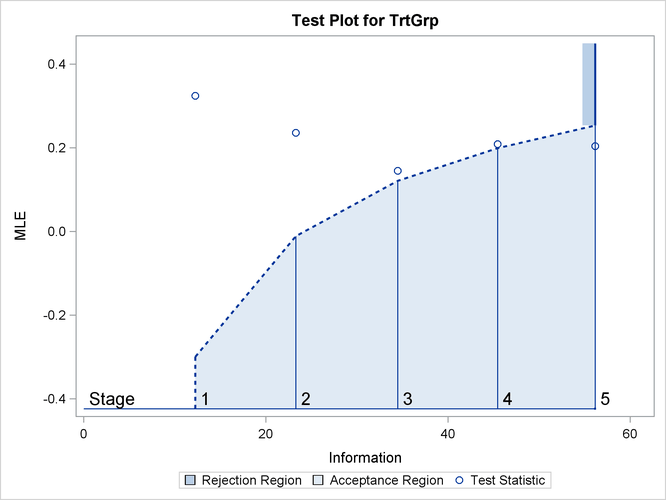After a trial is stopped, the Parameter Estimates table in Output 84.5.16 displays the stopping stage, parameter estimate, unbiased median estimate, confidence limits, and the p-value under the null hypothesis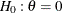. As expected, the p-value 0.0456 is not significant at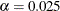level and the lower 97.5% confidence limit is less than the value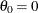. The p-value, unbiased median estimate, and confidence limits depend on the ordering of the sample space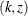, where k is the stage number and z is the standardized Z statistic.

Output 84.5.16: Parameter Estimates

Parameter Estimates
Stagewise Ordering
Parameter Stopping
Stage
MLE p-Value for
H0:Parm=0
Median
Estimate
Lower 97.5% CL
TrtGrp 5 0.204303 0.0456 0.234494 -0.03712

Since the test is accepted at stage 5, the p-value computed by using the default stagewise ordering can be expressed as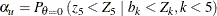where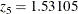is the test statistic at stage 5,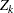is a standardized normal variate at stage k, and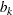is the upperboundary value in the standardized Z scale at stage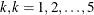.

With the RCI option, the Repeated Confidence Intervals table in Output 84.5.17 displays repeated confidence intervals for the parameter. For a one-sided test with an upper alternative hypothesis, since the upper acceptance repeated confidence limit 0.3924 at the final stage is less than the alternative reference 0.441833, the null hypothesis is accepted.

Output 84.5.17: Repeated Confidence Intervals

Repeated Confidence Intervals
_Stage_ Information
Level
Parameter
Estimate
Acceptance Boundary
Upper 89.94% CL
1 12.2601 0.32474 1.0656
2 23.2792 0.23560 0.6881
3 34.4879 0.14482 0.4653
4 45.4468 0.20855 0.4514
5 56.1607 0.20430 0.3924

With the PLOTS=RCI option, the Repeated Confidence Intervals Plot displays repeated confidence intervals for the parameter, as shown in Output 84.5.18. It shows that the upper acceptance repeated confidence limit at the final stage is less than the alternative reference 0.441833. This implies that the study accepts the null hypothesis at the final stage.

Output 84.5.18: Repeated Confidence Intervals Plot